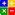# Ratio - Altman Z Score (for Private Firms)ALTMAN Z-SCORE (Private Firms):Online Z'-Score Calculator (for Private Firms)

The Revised Model (A Variation Adapted for Private Firms)

This score uses statistical techniques to predict a private firm's probability of failure using the following 8 variables from its' financial statements:

{For Z' Score the following 8 inputs are essential: {Current Assets; Current Liabilities; Total Liabilities; EBIT; Total Assets; Net Sales; Retained Earnings; Book Value of Equity}

The 5 financial ratios in the Altman Z'-Score for Private Firms and their respective weight factor is as follows:

 RATIO WEIGHTAGE A EBIT/Total Assets x. 3.107 -4 to +8.0 B Net Sales /Total Assets x 0.998 -4 to +8.0 C Book Value of Equity / Total Liabilities x 0.420 -4 to +8.0 D Working Capital/Total Assets x 0.717 -4 to +8.0 E Retained Earnings /Total Assets x0.847 -4 to +8.0

These ratios are multiplied by the weightage as above, and the results are added together.

Altman Z'-Score (for Private Firms) = (A x 3.107) + (B x 0.998) + (C x 0.420) + (D x 0.717) + (E x 0.847)

The Interpretation of Z' Score:

Z'-SCORE ABOVE 2.9 -  The company is deemed Safe based on the given financial figures only.
Z'-SCORE BETWEEN 1.23 and 2.9 - On Alert. This is the grey zone and one should exercise Caution.
Z'-SCORE BELOW 1.23 - Probability of Financial Embarassment is very High.

This website uses cookies to ensure you get the best experience on our website.